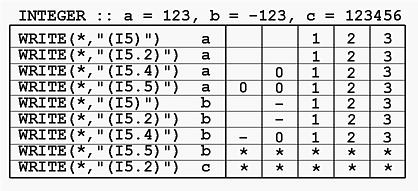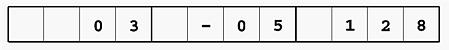# INTEGER Output: The I DescriptorThe Iw and Iw.m descriptors are for INTEGER output. The general form of these descriptors are as follows:

rIw    and     rIw.m

The meaning of r, w and m are:

• I is for INTEGER
• w is the width of field, which indicates that an integer should be printed with w positions.
• m indicates that at least m positions (of the w positions) must contain digits. If the number to be printed has fewer than m digits, leading 0s are filled. If the number has more than m digits, m is ignored and in this case Iw.m is equivalent to Iw.

Note that w must be positive and larger than or equal to m.

It is interesting to note that m can be zero! That is, no digits should be printed. In this case, if the number to be printed is non-zero, it will be printed as if Iw is used. However, if the number is zero, all w positions will be filled with spaces!

• r is the repetition indicator, which gives the number of times the edit descriptor should be repeated. For example, 3I5.3 is equivalent to I5.3, I5.3, I5.3.
• The sign of a number also needs one position. Thus, if -234 is printed, w must be larger than or equal to 4. The sign of a positive number is not printed.
• What if the number of positions is less than the number of digits plus the sign? In other words, what if a value of 12345 is printed with I3? Three positions are not enough to print the value of five digits. In this case, traditionally, all w positions are filled with *'s. Therefore, if you see a sequence of asterisks, you know your edit descriptor does not have enough length to print a number.### Examples

Let us look at the following example. There are three INTEGER variables a, b and c with values 123, -123 and 123456, respectively. In the following table, the WRITE statements are shown in the left and their corresponding output, all using five positions, are shown in the right.• The first line uses (I5) to print the value of 123. Thus, digits 1, 2 and 3 appear at the right end and two leading spaces are filled.
• The second line uses (I5.2) to print 123. This means of the five positions, two positions must contain digits. Since the value 123 has already had 3 digits. Therefore, .2 is ignored and the result is the same as that of the first line.
• The third line uses (I5.4) to print 123. Since m = 4, four positions must be filled with digits. Since 123 has only three digits, a leading 0 must be inserted. Thus, in the output, the five positions contain a space, 0, 1, 2 and 3.
• The fourth line uses I5.5 and therefore forces two 0s to be inserted.
• The fifth line uses (I5) to print -123. Since the number is negative, a minus sign is printed. The sixth line produces the same result as that of the fifth.
• The seventh line tells us that if leading zeros must be inserted, they are inserted between the minus sign and the number.
• The eighth line uses (I5.5) to print -123. This is not a good edit descriptor. I5.5 means to print a number using five positions and all five positions must be filled with digits. If the number is positive, there is no problem as shown on the fourth line. However, if the number is negative, there will be no position for the minus sign. As a result, all five positions are filled with asterisks, indicating a problem has occurred.
• The last line uses I5.2 to print 123456. The number has six digits and the number of positions to print this number is five. Thus, the given number 123456 cannot be printed completely and all five positions are filled with asterisks.
Consider the following example. The WRITE statement has three INTEGER variables and consequently the format must also have three I edit descriptors, one for each variable.

```INTEGER :: a = 3, b = -5, c = 128

WRITE(*,"(3I4.2)")  a, b, c
```
The edit descriptor is 3I4.2 and the format is equivalent to (I4.2,I4.2,I4.2) because the repetition indicator is 3. Therefore, each of the three INTEGER variables is printed with I4.2. Based on the discussion earlier, the result is the following: